# IBPS Clerk Mains Reasoning Questions 2019 (Day-08) High Level-New Pattern

Dear Aspirants, Reasoning Ability plays a crucial role in Banking and all other competitive exams. To enrich your preparation, here we have provided New Pattern Reasoning Questions for IBPS Clerk Mains. Candidates those who are going to appear in IBPS Clerk Mains can practice these questions daily and make your preparation effective.

[WpProQuiz 4732]

(Directions 1–5): Study the following information carefully and answer the questions given below it.

^ – South

% – East

@ – North

# – West

\$ – Either 3 metre or 5 metre.

£ – Either 4 metre or 8 metre.

¥ – Either 6 metre or 9 metre.

i.e. P ^ Q means P is south of Q.

P ^% Q means P is South-East of Q.

P ^\$ Q means P is either 3 metre or 5 metre south of Q.

Given condition:

(i) P @¥ U

(ii) T ^£ S

(iii) Q %£ P

(iv) U #\$ T

(v) R ^\$ Q

(iv) S #\$ R

(vii) V ^ Q

(viii) Distance between V and Q is 17 metre.

(ix) Length of UT is more than TS.

1) What is the position of T with respect V?

a) East

b) North-East

c) South

d) North-West

e) Can’t be determined.

2) What is shortest distance between S to U?

a) 3m

b) √41m

c) 2√7m

d) 7m

e) Can’t be determined.

3) What is the position of R with respect with respect to P?

a) South

b) West

c) South-East

d) South-West

e) Can’t be determined.

4) If D #\$ S, M ^% U and M ^£ D, then which of the following statement is definitely not true?

a) M is north-west of point V.

b) Shortest distance between TM is 5m.

c) D is 6m west of R.

d) P is north-west of M.

e) All the given statement is true.

5) If Sam starts walking towards south from point U and after walking 5m takes a left turn and walks another 5m, again he takes a right turn and walks 3m to reach point K, then what is distance between V and K?

a) 2√3m

b) 4m

c) √5m

d) 3m

e) 6m

(Directions 6–10): Study the following information carefully and answer the questions given below it.

In a certain code language some statements are coded as follow:

“Move Down Above Major Kind” is coded as “[email protected] 4%F 4#F [email protected] 6%Q”.

“High None Voltage Taken Believe” is coded as “5%M 4&F [email protected] 7#F 5%F”.

“Mobile Deep Hold Heavy Round” is coded as “3#F 5%C 4%X 5%O [email protected]”.

“Build Better Nation Together Nature” is coded as “6#M 3%C 5%Q 9#F 5#Q”.

6) How “Major Deep Nature” is coded in given code language?

a) [email protected] 5%O 5%Q

b) 9#F 6%Q 5%O

c) 6#M 3#F 5%O

d) [email protected] 5%C 3%C

e) None of these

7) How “Always Build Calculate Develop” is coded in same code language?

a) 3%C 3%G 2&F 7#R

b) 2&F 6#Q [email protected] 3%C

c) 3%C 5%P [email protected] 6&Q

d) 6#O 2&F 3%C 7%R

e) None of these

8) If “Put Above Night Rocket Science” is coded as “__4#F__3#F__” then, what is the missing code?

c) 3%U 7#S 5%S

d) [email protected] 7%S 3#R

e) None of these

9) Which of the following statement is coded as “[email protected] 3%Q 4%F” is coded in same code language?

b) Maker More Motion

d) Either A or C

10) Which of the following statement is coded as “4%X 5%S 6#M” is coded in same code language?

a) Heavy Point Motion

b) Maker Heavy Point

c) Either A or B

d) Heavy Bond Cast

e) None of these

(Directions 1–5):

We have:

• P is either 6m or 9m north of U.
• T is either 4m or 8m south of S.
• Q is either 4m or 8m east of P.
• U is either 3m or 5m west of T.
• R is either 3m or 5m south of Q.
• S is either 3m or 5m west R.
• V is south of Q.

Based on above given information we have: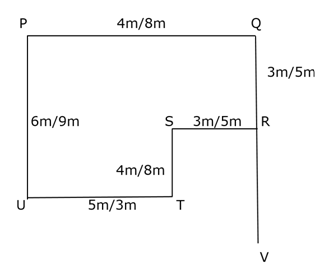Again, we have:

• Length of UT is more than TS, that means UT must be 5m and TS must be 4m, as PQ more than UT that means PQ = 8m.

Thus, SR = 3m.

• As, ST = 4m, that means Possible length of PU be (3, 4) or (5, 4), that means only possible length of PU and QR must be 9m and 5m respectively.
• Distance between V and Q is 17 meter, as QR = 5m.

Thus, RV = (17 – 5) = 12m.

Based on above given information we final arrangement as follow: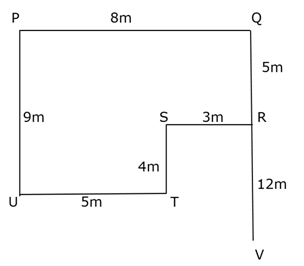We have:

Clearly, T is North-West of V.

We have: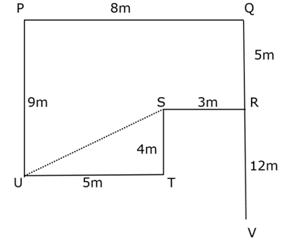Clearly, UT = 5m & ST = 4m

Thus, US = √(52 + 42) = √41 metre

Hence, option B is correct choice.

We have:

Clearly, R is south-east of P.

Hence, option C is correct choice.

We have:

Since, M is south-east of U, that means DS = 3m and DM = 8m.

From above given information we have: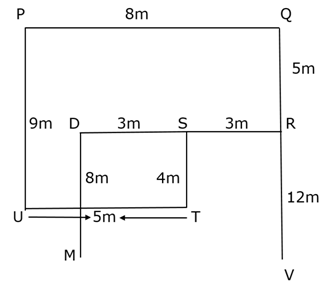Clearly, all the given information is true.

Hence, option E is correct choice.

We have: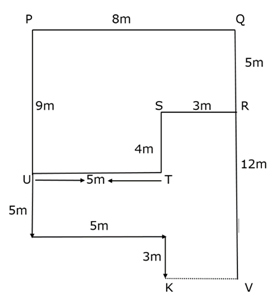Clearly, distance between KV = 3m.

Hence, option D is correct choice.

(Directions 6–10):

We have: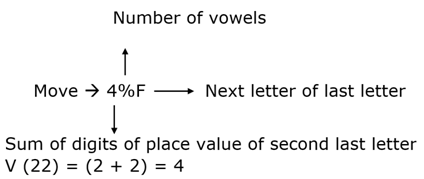Above statements are coded as follow:

• If number of vowels is one then coded as ‘@’, if number of vowels is two then coded as ‘%’, if number of vowels is three then coded as ‘#’ and if number of vowels is four then coded as ‘&’.
• If last letter is consonant then change letter to previous letter and if last letter is vowel then change letter to next letter of English alphabetical series.
• Add sum of digits of place value second last letter of English alphabetical series.

For example:

If second last letter is N(14) = (1 + 4) = 5

Similarly,

Taken -> 5%M

We have:

Clearly, Major -> 6%Q

Deep -> 5%O

Nature -> 9#F

Hence, option B is correct choice.

We have:

Always -> 7%R

Build -> 3%C

Calculate -> 2&F

Develop ->6#O

Hence, option D is correct choice.

We have:

Above -> 4#F

Science -> 2#F

Put -> [email protected]

Night -> [email protected]

Rocket -> 5%S

Thus, required missing code be “[email protected] [email protected] 5%S”.

Hence, option B is correct choice.

We have:

[email protected] -> Hold

3%Q -> Your

Hence, option D is correct choice.

We have:

4%X -> Heavy

5%S -> Point

6#M -> Motion

Hence, option A is correct choice.